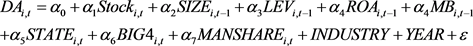﻿ 企业战略、高管薪酬激励与盈余管理

# 企业战略、高管薪酬激励与盈余管理Corporate Strategy, Executive Compensation Incentives and Earnings Management

Abstract: Taking China’s A-share listed companies from 2006 to 2017 as a research sample, we discuss two kinds of executive compensation incentives—the impact of monetary incentives and equity incen-tives on earnings management, and further explore the impact differences. The results show that both monetary incentives and equity incentives for executives will increase the degree of earnings management; compared with monetary incentives, executive equity incentives have a stronger im-pact on corporate earnings management; compared to companies that adopt cost-leading strategies enterprises adopting a differentiated strategy will strengthen the positive correlation between monetary incentives and corporate earnings management.

1. 引言

2. 理论分析与研究假设

2.1. 高管薪酬与盈余管理

2.1.1. 高管货币激励对盈余管理的影响

H1：对高管进行货币激励会提高企业盈余管理程度。

2.1.2. 高管股权激励对盈余管理的影响

H2：对高管进行股权激励会提高企业盈余管理程度。

2.1.3. 公司战略对高管激励与盈余管理关系的影响

H3a：相较于采取成本领先型战略的企业，采取差异化战略的企业会强化对高管进行货币激励与企业盈余管理之间的正相关关系。

H3b：相较于采取成本领先型战略的企业，采取差异化战略的企业会强化对高管进行股权激励与企业盈余管理之间的正相关关系。

3. 研究设计

3.1. 数据来源与样本选择

3.2. 变量选取

3.2.1. 公司战略的衡量

STR变量的构建方法如下所示，首先将上述每个变量取过去5年的平均值，然后按将上述六个变量在每一个“年度—行业”样本中进行排序分为5组。其中，对于(1)、(2)、(4)、(5)这四个变量，最小组赋值为1，第二小组赋值为2，依次类推，最大组赋值为5。对于(3)、(6)这两个变量，最小组赋值为5，第二小组赋值为4，依次类推，最大组赋值为1。最后将这六个变量的得分加起来作为STR变量的取值。STR越大，公司越倾向于采取进攻性战略，STR越小，公司越倾向于采取防御性战略。

3.2.2. 盈余管理程度的度量

$\frac{T{A}_{i,t}}{{A}_{i,t-1}}={\alpha }_{1}\frac{1}{{A}_{i,t-1}}+{\alpha }_{2}\frac{\Delta RE{V}_{i,t}}{{A}_{i,t-1}}+{\alpha }_{3}\frac{PP{E}_{i,t}}{{A}_{i,t-1}}+{\epsilon }_{i,t}$ (1)

$ND{A}_{i,t}={\stackrel{^}{\alpha }}_{1}\frac{1}{{A}_{i,t-1}}+{\stackrel{^}{\alpha }}_{2}\frac{\Delta RE{V}_{i,t}-\Delta RE{C}_{i,t}}{{A}_{i,t-1}}+{\stackrel{^}{\alpha }}_{3}$ (2)

 (3)

1) 两种高管激励措施的衡量

a) 高管货币激励指标

b) 高管股权激励的指标

2) 控制变量

3.3. 模型构建

$\begin{array}{l}D{A}_{i,t}={\alpha }_{0}+{\alpha }_{1}Pa{y}_{i,t}+{\alpha }_{2}SIZ{E}_{i,t-1}+{\alpha }_{3}LE{V}_{i,t-1}+{\alpha }_{4}RO{A}_{i,t-1}+{\alpha }_{4}M{B}_{i,t-1}\\ +{\alpha }_{5}STAT{E}_{i,t}+{\alpha }_{6}BIG{4}_{i,t}+{\alpha }_{7}MANSHAR{E}_{i,t}+INDUSTRY+YEAR+\epsilon \end{array}$ (4)(5)

$\begin{array}{l}D{A}_{i,t}={\alpha }_{0}+{\alpha }_{1}Pa{y}_{i,t}+{\alpha }_{2}Pa{y}_{{}_{i,t}}^{\text{*}}ST{R}_{i,t}+{\alpha }_{3}ST{R}_{i,t}+{\alpha }_{4}SIZ{E}_{i,t-1}+{\alpha }_{5}LE{V}_{i,t-1}+{\alpha }_{6}RO{A}_{i,t-1}\\ +{\alpha }_{7}M{B}_{i,t-1}+{\alpha }_{8}STAT{E}_{i,t}+{\alpha }_{9}BIG{4}_{i,t}+{\alpha }_{10}MANSHAR{E}_{i,t}+INDUSTRY+YEAR+\epsilon \end{array}$ (6)

$\begin{array}{l}D{A}_{i,t}={\alpha }_{0}+{\alpha }_{1}Stoc{k}_{i,t}+{\alpha }_{2}Stoc{k}_{{}_{i,t}}^{\text{*}}ST{R}_{i,t}+{\alpha }_{3}ST{R}_{i,t}+{\alpha }_{4}SIZ{E}_{i,t-1}+{\alpha }_{5}LE{V}_{i,t-1}+{\alpha }_{6}RO{A}_{i,t-1}\\ +{\alpha }_{7}M{B}_{i,t-1}+{\alpha }_{8}STAT{E}_{i,t}+{\alpha }_{9}BIG{4}_{i,t}+{\alpha }_{10}MANSHAR{E}_{i,t}+INDUSTRY+YEAR+\epsilon \end{array}$ (7)

4. 实证分析

4.1. 描述性统计

4.2. 回归分析

1) 对假设1、假设2的检验。即高管货币激励对企业盈余管理的影响和高管股权激励对企业盈余管理的影响。如下表3所示。Table 3. Influence of executive monetary incentives and equity incentives on corporate earnings managementTable 4. The impact of different corporate strategies on executives’ monetary incentives or equity incentives and corporate earnings management

2) 对假设3(a)、3(b)的检验。验证相较于采取成本领先型战略的企业，采取差异化战略的企业会强化对高管进行货币激励或股权激励与企业盈余管理之间的正相关关系。从表4中第一列可以看出，Pay与Strindx交乘项的系数在10%的置信水平下显著为正，这说明相较于采取成本领先型战略的企业，采取差异化战略的企业会强化对高管进行货币激励与企业盈余管理之间的正相关关系，从而验证了假设3a。从表4第二列可以看出Stock与Strindx交乘项的系数在10%的置信水平下显著为正，这说明相较于采取成本领先型战略的企业，采取差异化战略的企业会强化对高管进行股权激励与企业盈余管理之间的正相关关系，从而验证了假设3b。可能的原因在于，相较于采取成本领先型战略的企业而言，采取差异化战略的企业更倾向于不断寻求新的创新产品和市场机会，这就需要大量投资于研发活动，从而增加损失风险。这说明在差异化公司战略的情况下，高管面临业绩激励时进行盈余管理的动机会更大，更会采取措施进行盈余管理。

4.3. 稳健性检验

5. 研究结论及建议

 Dye, R.A. and Sridhar, S.S. (2016) Hedging Executive Compensation Risk through Investment Banks. The Accounting Review, 91, 1109-1138.
https://doi.org/10.2308/accr-51291

 Hui, K.W. and Matsunaga, S.R. (2015) Are CEOs and CFOs Rewarded for Disclosure Quality? The Accounting Review, 90, 1013-1047.
https://doi.org/10.2308/accr-50885

 Flor, C.R., Frimor, H. and Munk, C. (2014) Options in Compensation: Promises and Pitfalls. Journal of Accounting Research, 52, 703-732.
https://doi.org/10.1111/1475-679X.12049

 王珏玮, 唐建新, 孔墨奇. 公司并购、盈余管理与高管薪酬变动[J]. 会计研究, 2016(5): 56-62.

 黄文伴, 李延喜. 管理者薪酬契约与企业盈余管理程度关系[J]. 科研管理, 2011, 32(6): 133-139.

 潘红洋. 最新盈余管理文献综述[J]. 经济论坛, 2015(8): 127-129.

 童娜琼, 岑维, 谢思东. 有财务背景的当地独立董事和真实盈余管理[J]. 财会月刊, 2015(30): 3-9.

 Rajagopalan, N. and Spreitzer, G.M. (1997) Toward a Theory of Strategic Change: A Multi-Lens Perspective and Integrative Framework. Academy of Management Review, 22, 48-79.
https://doi.org/10.5465/amr.1997.9707180259

 Efendi, J., Srivastava, A. and Swanson, E.P. (2007) Why Do Corporate Managers Misstate Financial Statements? The Role of Option Compensation and Other Factors. Journal of Financial Economics, 85, 667-708.
https://doi.org/10.1016/j.jfineco.2006.05.009

 孙健, 王百强, 曹丰, 刘向强. 公司战略影响盈余管理吗?[J]. 管理世界, 2016(3): 160-169.

 Bentley, K.A., Omer, T.C. and Sharp, N.Y. (2013) Business Strategy, Financial Reporting Irregularities, and Audit Effort. Contemporary Accounting Research, 30, 780-817.
https://doi.org/10.1111/j.1911-3846.2012.01174.x

 李广森. 财务门槛、盈余管理与公司业绩[J]. 财会月刊, 2017(20): 12-20.

Top